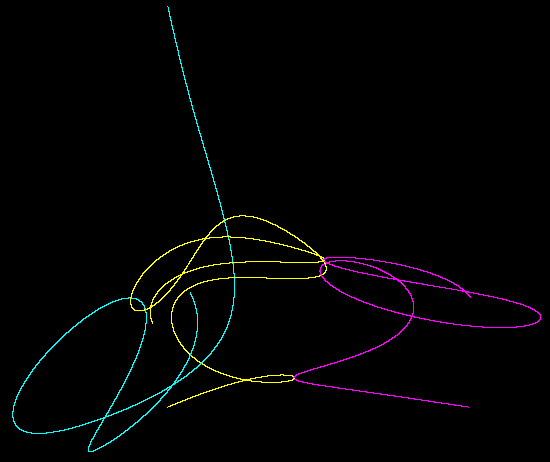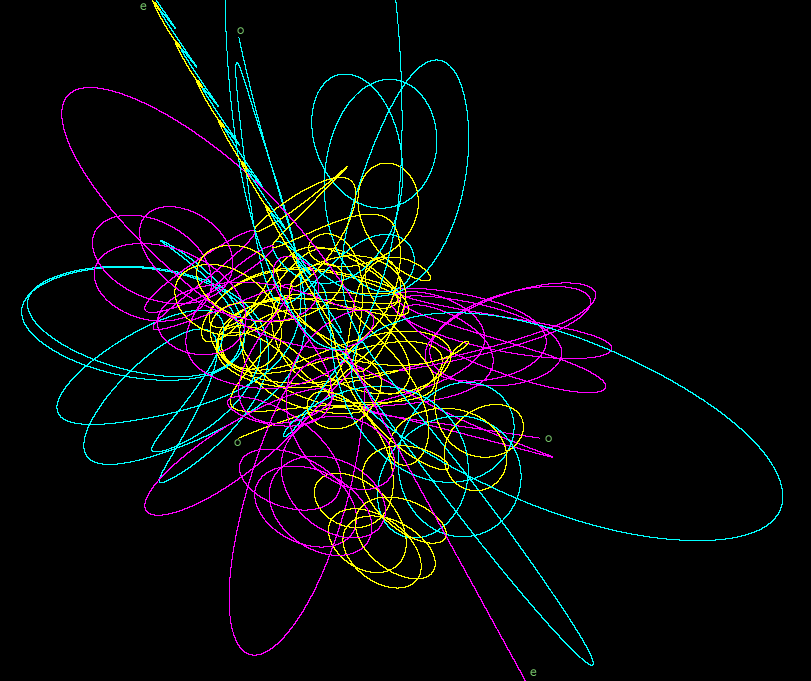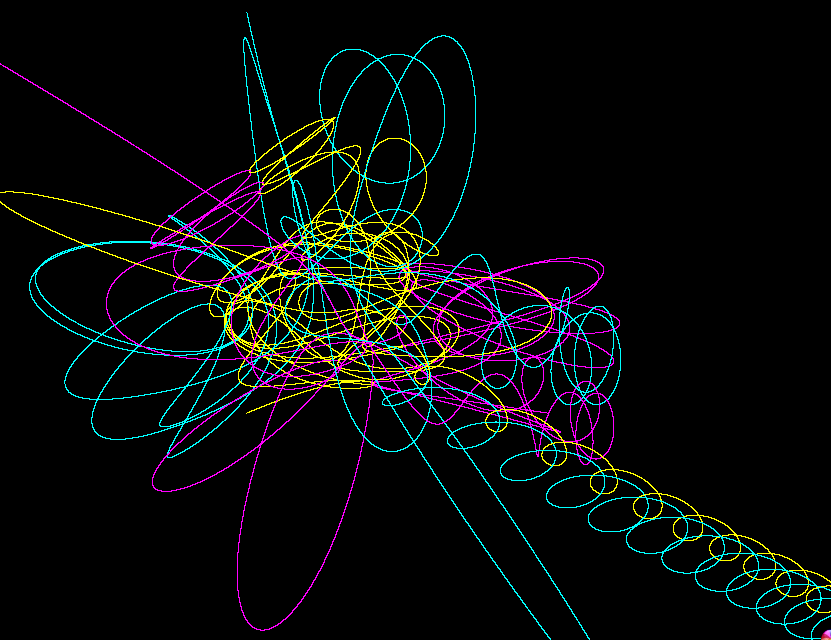Here are the good accurate pictures; below are introductions to the ideas.

Here is an amusing little three body problem related by Diacu & Holmes “Celestial Encounters” (ISBN = 0691027439). Three point masses begin at rest at the vertices of a right 3-4-5 triangle. Their masses are proportional to the length of the opposite side. Naturally, they remain in the initial plane of the triangle. Here (a68) is a too simple version of code to compute their progress. (See note on C dialect, and Algol68.) It is drastically off by t = 10 for Δt = 10−6. Practically this problem requires both variable Δt and some higher order numerical method. This C code uses a variable Δt and writes a file that this Java program can plot. Here are some fragmentary thoughts on the math. Here are the code and pictures computed with the fourth order Runga-Kutta which makes the best and most accurate pictures.

Below is a picture of the calculation to t=10:Here are the trails unto expulsion:.

The image below is computed by this code and drawn by this. The initial positions are marked with “o” and the places and the picture’s edge where the particles leave for the last time are marked with “e”. The calculation took about 1,750,000 steps. r/dx was limited to about 1200.And again with r/dx at about 4000.A lone mass m, at radius r with velocity v has potential energy −m(12-m)2/(12r) and kinetic energy mv2/2.
(define (e m x y xd yd) (let ((v2 (+ (sq xd) (sq yd)))(sq (lambda (x)(* x x)))(r (sqrt (+ (sq x) (sq y))))) (- (/ (* m v2) 2) (/ (* m (sq (- 12 m))) (* 12 r)))))